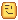# LookVector not working properly?

I’m trying to make a door thing (with 2 door unions) that tween open and closed.

However, as seen in this video, it’s not working properly

Here’s the output from what I gave as the lookVector argument to create the CFrame for Part1, and what the resulting lookVector was:

0, 0, 4 - what I set it to
-0.0678792596, 0.406093419, -0.911307096 - what it is

Here’s my code:

``````local vector = Vector3.new(0,0,5)
local moveVector1 = vector*part1.CFrame.lookVector.unit
local moveVector2 = vector*part2.CFrame.lookVector.unit
local newLook1 = part1.CFrame.lookVector - moveVector1
local newLook2 = part2.CFrame.lookVector + moveVector2

local newCFrame1 = CFrame.new(part1.CFrame.p-moveVector1,newLook1)
local newCFrame2 = CFrame.new(part2.CFrame.p+moveVector2,newLook2)

print(newLook1,newCFrame1.lookVector)
``````

ThanksThose doors look unioned, is it possible that they are built off axis?

2 Likes

A CFrame’s lookVector is the forwards component of the rotation matrix, but the second argument to the CFrame constructor is the point in 3D space the CFrame should be rotated to look at.

So if you create a CFrame with `CFrame.new(p1, p2)`, its lookVector will be `(p2 - p1).unit`. So those two values being different is to be expected. To create a CFrame from position and lookVector directly you can do `CFrame.new(p1, p1+lookVector)`.

You’re using the `*` operator on two Vector3s. This does componentwise multiplication, which is almost never what you want (I’m not even sure about the ‘almost’). In this case you want `part1.CFrame:vectorToWorldSpace(vector)`, which transforms the vector by applying the rotation but not the translation.

Looks like what you want to happen is that the parts end up moved by a vector, which is relative to the part’s rotation, and not have their rotation changed at all, which you can just do like this (assuming I got the order of CFrame multiplication right):

``````local vector = Vector3.new(0,0,5)
local newCFrame1 = part1.CFrame * CFrame.new(vector)
local newCFrame2 = part2.CFrame * CFrame.new(-vector)
``````
5 Likes

It works - it just doesn’t make sense to me though.

Multiplication is supposed to multiply things, not just add 5 studs to a CFrame. Could you explain how this works a bit more?

The `*` operator for CFrames is not multiplication.

http://wiki.roblox.com/index.php?title=CFrame#CFrame_.2A_CFrame

1 Like

Still kinda confused (the usual inconsiderate wiki wording hehe), but thanks.

1 Like

That’s because he was being a pedant. Everyone always simply calls composition of CFrames / Transformation Matrices “multiplication”, especially in the context of game creation. What name you use does nothing to answer your question.

Multiplication is supposed to multiply things, not just add 5 studs to a CFrame. Could you explain how this works a bit more?

To answer your question, the more important point is that “multiplication” (or whatever you choose to call the `*` operator) does not mean the same thing for every type. Yes, for integers and real numbers, you have the familiar definition of multiplication, but that definition isn’t very useful for more non-trivial composite data types like Complex Numbers, or Matrices–which is what a CFrame really is.

At the end of the day, if you want the TL;DR: You should think of multiplication for CFrames being more similar to addition for normal numbers. Multiplying a CFrame onto a base CFrame “adds” the transformation that that CFrame represents to the base one.

This image is should be really helpful (taken from here, though I wouldn’t recommend that page, I think it’s a bit too technical for someone without a very strong math background to grok):

9 Likes

I’m reading that page now and it’s quite interestingThank you!